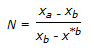Courses

# Test: Mass Transfer - 7

## 25 Questions MCQ Test Mass Transfer | Test: Mass Transfer - 7

Description
This mock test of Test: Mass Transfer - 7 for Chemical Engineering helps you for every Chemical Engineering entrance exam. This contains 25 Multiple Choice Questions for Chemical Engineering Test: Mass Transfer - 7 (mcq) to study with solutions a complete question bank. The solved questions answers in this Test: Mass Transfer - 7 quiz give you a good mix of easy questions and tough questions. Chemical Engineering students definitely take this Test: Mass Transfer - 7 exercise for a better result in the exam. You can find other Test: Mass Transfer - 7 extra questions, long questions & short questions for Chemical Engineering on EduRev as well by searching above.
QUESTION: 1

Solution:
QUESTION: 2

Solution:
QUESTION: 3

### Steam distillation is used to

Solution:
QUESTION: 4

Freundlich equation applies to the adsorption of solute from

Solution:
QUESTION: 5

For ethanol-water system, the lowering of distillate quality from 95% to 92% will cause __________ plate requirement.

Solution:
QUESTION: 6

The apex of an equilateral-triangular coordinate (in ternary liquid system) represents a/an

Solution:
QUESTION: 7

The diffusivity (D) in a binary gas mixture is related to the pressure (P) as

Solution:
QUESTION: 8

A slurry is to be dried to produce flaky solid. Which dryer would you recommend ?

Solution:
QUESTION: 9

Which of the following psychrometric processes is followed by water vapour laden unsaturated air, when it is passed through solid or liquid adsorbent ?

Solution:
QUESTION: 10

In gas-liquid contact operation, the number of ideal stages,. This is true when the stripping factor 'S' is

Solution:
QUESTION: 11

The cooling effect in a cooling tower can not be increased by

Solution:
QUESTION: 12

The value of NA/(NA + NB) for steady state molecular diffusion of gas 'A' through non-diffusing gas 'B' is

Solution:
QUESTION: 13

The humid volume may be increased by increasing the

Solution:
QUESTION: 14

Pick out the wrong statement

Solution:
QUESTION: 15

When the mixture to be distilled has a very high boiling point and the product material is heat sensitive, the separation technique to be used is __________ distillation.

Solution:
QUESTION: 16

Large scale drying of wheat is done in a __________ dryer.

Solution:
QUESTION: 17

Azeotropic distillation is employed to separate

Solution:
QUESTION: 18

The drying time between fixed moisture content within diffusion controlled 'falling rate period' is proportional to (assuming that drying occurs from all surfaces of the solid) (where, T = thickness of the solid )

Solution:
QUESTION: 19

At the boiling point of the liquid at the prevailing pressure, the saturated absolute humidity becomes

Solution:
QUESTION: 20

Use of natural draft cooling tower is practised, when the air has low

Solution:
QUESTION: 21

Pressure drop through plate tower as compared to that through packed tower, for the same duty will be

Solution:
QUESTION: 22

Dimension of mass diffusivity is the same as that of

Solution:
QUESTION: 23

Dorr thickener is an equipment used for

Solution:
QUESTION: 24

Steam distillation is used to separate

Solution:
QUESTION: 25

In distillation where q is defined as the moles of liquid flow in the stripping section per mole of feed introduced, for saturated liquid feed

Solution: